#Function Repository Resource:

# PhiNumberSystem

Get a list of powers of the golden ratio which sum to a given integer

Contributed by: Wolfram Staff
 ResourceFunction["PhiNumberSystem"][n] gives the list {x1,x2,…,xk} such that ϕx1 + ϕx2 + … + ϕxk = n, where ϕ is GoldenRatio.

## Details and Options

The list consists of nonconsecutive distinct integers that may be 0 or negative.
The input must be a positive integer.

## Examples

### Basic Examples

This gives the golden ratio powers needed to represent 10:

 In:=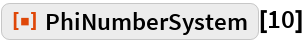Out=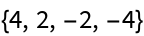Indeed, the sum of those powers of ϕ is 10:

 In:=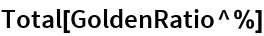Out=In:=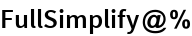Out=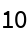### Scope

Here are the representations of the first 20 integers:

 In:=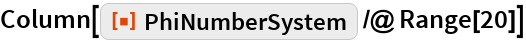Out=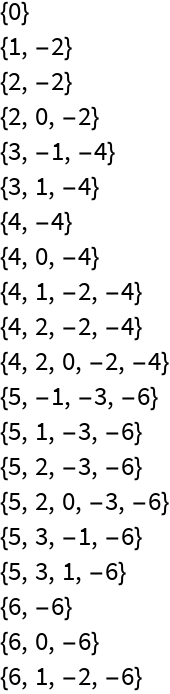### Neat Examples

The sequence of lists seems to be fractal:

 In:=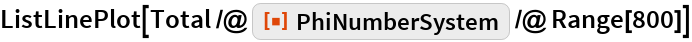Out=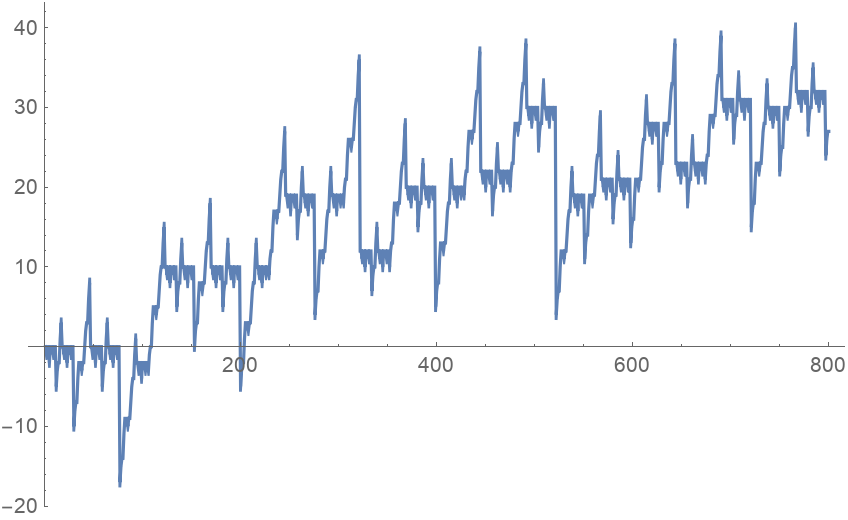## Requirements

Wolfram Language 11.3 (March 2018) or above